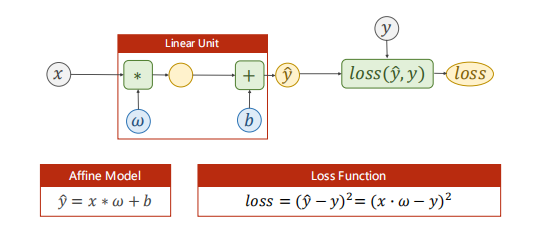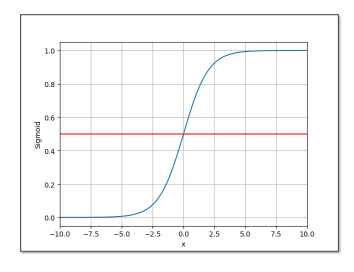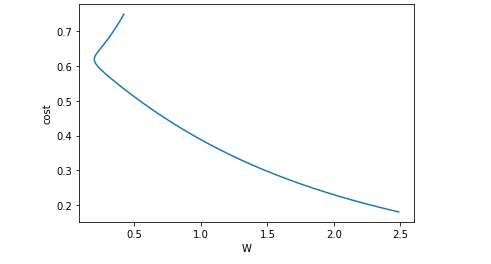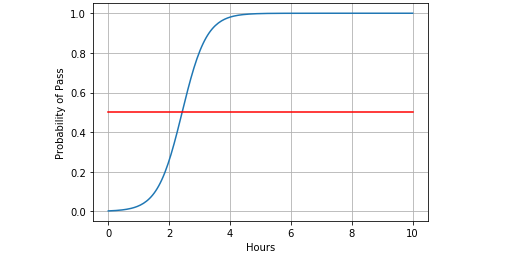## 回顾

之前我们讨论过一个线性模型：上述模型预测出的$\hat{y} \in R$属于一个连续的空间内，我们称这类任务为回归任务。但是很多的机器学习任务要求我们去分类，比如给动物图片分类、给手写数字分类。对于分类任务，它预测出来的结果属于一个离散的集合，例如手写数字分类的结果集合为$y \in \left[ 0,1,2,3,4,5,6,7,8,9 \right]$。对于分类任务，用回归模型去预测是不合适的，因为这些类别之间并没有连续空间中的数值大小的含义（不能说某个类别大于或小于某个类别如猫大于狗）。在分类问题中，分类模型输出一个概率分布，再在所有类别的概率值（0 ~ 1）中找到最大值就是预测结果了。另外要注意的是，逻辑回归不是回归，它常用于分类问题，只是名字易让人误会。

接下来，不妨用一个二分类任务来进行讨论。问题描述为每周学习时间与是否通过期末考试的关系。

## 输出映射$$\hat{y} = \sigma(x * w + b)$$ ![](https://hexoblog-1257022783.cos.ap-chengdu.myqcloud.com/Logistic/20200426013527455.png) 还有其他的Sigmoid函数： ![](https://hexoblog-1257022783.cos.ap-chengdu.myqcloud.com/Logistic/20200426111715624.png) 事实上，只要某函数满足单调增、有极限、为饱和函数（在超过一定阈值之后函数值变化微小） ## 损失函数  对于线性模型的损失函数： $$loss = (\hat{y} - y)^2 = (x \cdot w - y)^2$$ 是计算预测值与真实值的差别的函数，是有实际意义的。但是，在分类问题中的输出为一个概率分布，不再是简单的几何1度量之间的差别，因此该损失函数不再适用。转而用计算两个分布之间差异的函数，其中交叉熵损失函数事一个常用的方法： $$Loss(\hat{y}^{(i)}, y^{(i)}) = - \sum_{j=1}^{q} y_{j}^{(i)} \log \hat{y}_{j}^{(i)}$$ 由于在上式中，向量$y^{(i)}$只有一个元素为 1 其余全为 0，于是该式即为：$Loss(\hat{y}^{(i)}, y^{(i)}) = -\log \hat{y}_{j=nozero}^{(i)}$。也就是说，交叉熵值关心对正确类别的预测概率，因为只要其值足够大，就可以确保分类结果的正确性。当然，遇到一个样本有多个标签时，例如一张图片中既有猫也有狗时，我们并不能做这一步简化。 > 参数释义： > * $\hat{y}^{(i)}$ 是一个预测概率分布向量 > * $\hat{y}_{j}^{(i)} \Leftrightarrow P(Class_j)$ > * $y^{(i)}$ 一个维度为类别数且只有一个 1 其余为 0 的分布向量 > * $y^{(i)}_{j}$ 是向量 $y^{(i)}$ 中非 0 即 1 的元素 > * $q$ 为类别数量 对于一个二分类问题，损失函数为(BCE)： $$loss = -(y \log \hat{y} + (1-y)\log (1 - \hat{y}))$$ > 参数释义： > * $\hat{y} \Leftrightarrow P(Class_i)$ > * $y \Leftrightarrow (0 \text{ } or \text{ } 1)$ 小批量的二分类损失函数为： $$loss = -\frac{1}{N} \sum_{n=1}^{N} \left(y_n \log \hat{y}_n + (1-y_n)\log (1-\hat{y}_n) \right)$$ ![损失计算实例](https://hexoblog-1257022783.cos.ap-chengdu.myqcloud.com/Logistic/20200426014329153.png) ## 逻辑回归模型的实现

## 收敛图像## 测试